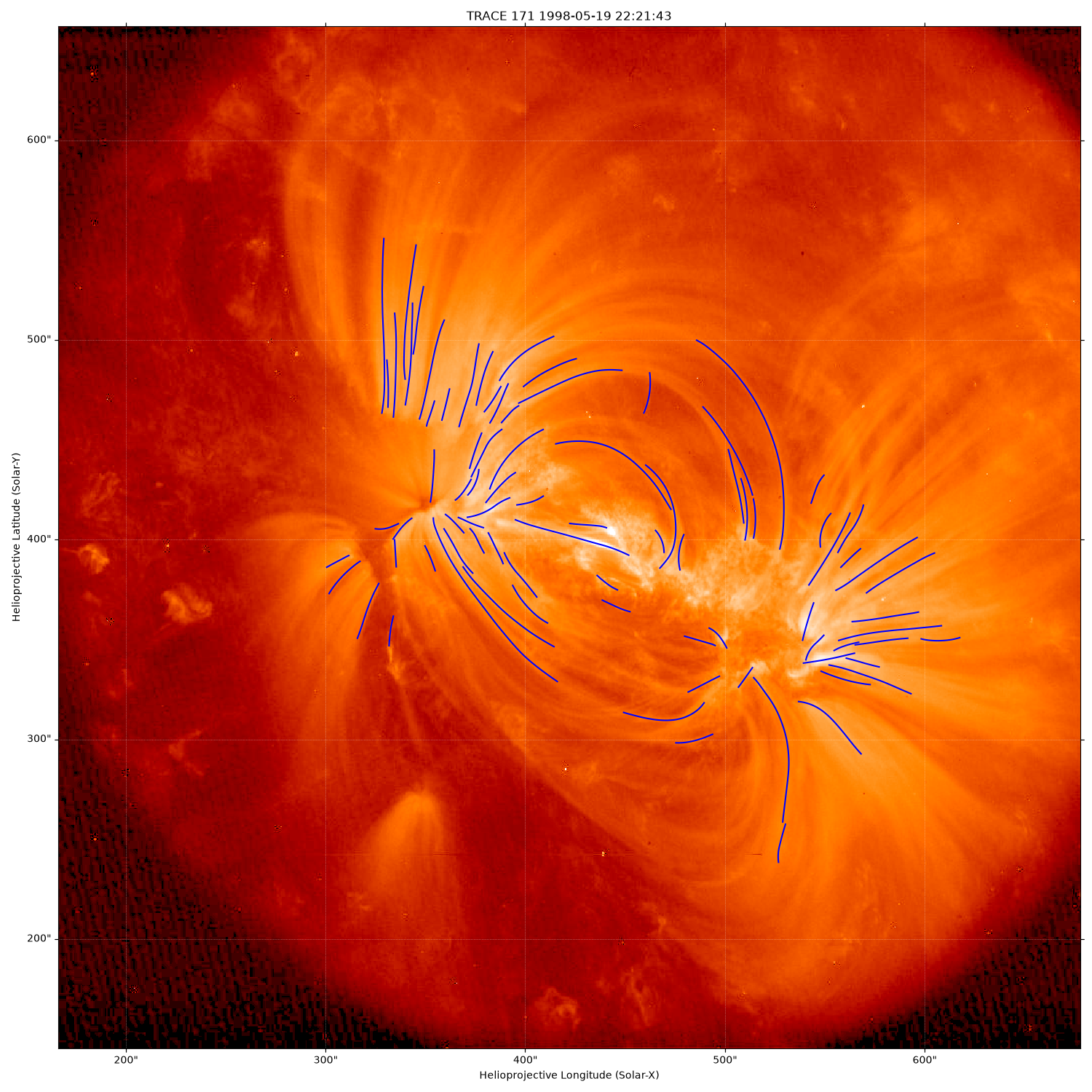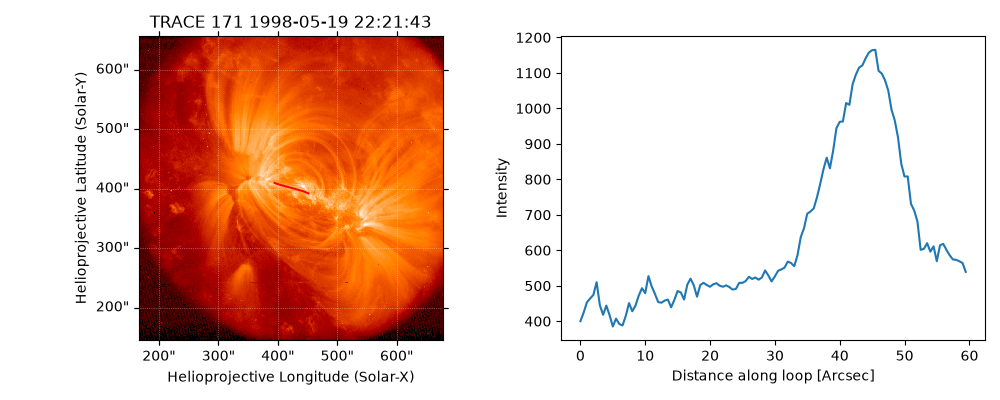# Tracing Coronal Loops#

This example traces out the coronal loops in a FITS image using `occult2`. In this example we will use the settings and the data from Markus Aschwanden’s tutorial on his IDL implementation of the `OCCULT2` algorithm, which can be found here. The aim of this example is to demonstrate that `occult2` provides similar results compared to the IDL version.

```import matplotlib.pyplot as plt
import numpy as np
import sunpy.map
from astropy import units as u
from astropy.io import fits

import sunkit_image.trace as trace
```

We will be using `astropy.io.fits.open` to read the FITS file from the tutorial website and read in the header and data information.

```hdu = fits.open("http://data.sunpy.org/sunkit-image/trace_1998-05-19T22:21:43.000_171_1024.fits")
# We can now make this into a `sunpy.map.GenericMap`. There is currently not an instrument specific
# class for the TRACE instrument.
# We can now plot the map, of which we can see coronal loops.
trace_map.plot()
``````INFO: Missing metadata for solar radius: assuming the standard radius of the photosphere. [sunpy.map.mapbase]
For frame 'heliographic_stonyhurst' the following metadata is missing: dsun_obs,hglt_obs,hgln_obs
For frame 'heliographic_carrington' the following metadata is missing: dsun_obs,crln_obs,crlt_obs

obs_coord = self.observer_coordinate

<matplotlib.image.AxesImage object at 0x7f64b6d6a590>
```

Now the loop tracing will begin. We will use the same set of parameters as in the IDL tutorial. The lowpass filter boxcar filter size `nsm1` is taken to be 3. The minimum radius of curvature at any point in the loop `rmin` is 30 pixels. The length of the smallest loop to be detected `lmin` is 25 pixels. The maximum number of structures to be examined `nstruc` is 1000. The number of extra points in the loop below noise level to terminate a loop tracing `ngap` is 0. The base flux and median flux ratio `qthresh1` is 0.0. The noise threshold in the image with respect to median flux `qthresh2` is 3.0 . For the meaning of these parameters please consult the OCCULT2 article.

```loops = trace.occult2(trace_map.data, nsm1=3, rmin=30, lmin=25, nstruc=1000, ngap=0, qthresh1=0.0, qthresh2=3.0)
```

`occult2` returns a list, each element of which is a detected loop. Each detected loop is stored as a list of `x` positions in image pixels, and a list of `y` positions in image pixels, of the pixels traced out by OCCULT2. Now plot all the detected loops on the original image, we convert the image pixels to to world coordinates to be plotted on the map.

```fig = plt.figure()
ax = plt.subplot(projection=trace_map)
trace_map.plot()
# We can now plot each loop in the list of loops. We plot these in world coordinates, converting them
# through the `pixel_to_world` functionality which converts the pixel coordinates to coordinates (in arcsec)
# on the `trace_map`.
for loop in loops:
loop = np.array(loop)  # convert to array as easier to index `x` and `y` coordinates
coord_loops = trace_map.pixel_to_world(loop[:, 0] * u.pixel, loop[:, 1] * u.pixel)
ax.plot_coord(coord_loops, color="b")

plt.show()
```Total running time of the script: (0 minutes 30.546 seconds)

Gallery generated by Sphinx-Gallery